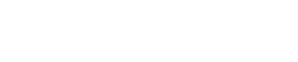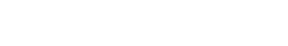## CHEMISTRY PAPER 1 - 2017 KITUI MOCK EXAMINATION

1. The table below shows the pH values of some solutions.
 Solutions J K L M N pH 6 13 2 10 7

1. Which solution is likely to be:
1. Potassium hydroxide.  (1 mark)
2. Lemon juice.               (1 mark)
2. Explain why a solution of hydrogen chloride gas in methylbenzene was identified as N.  (1 mark)
3. Compare the electrical conductivity of solution J and L.    (1 mark)
1. Name the process that takes place when:
1. Sulphur is heated with natural rubber.   (1 mark)
2. Fats or oils are hydrolysed using an alkali.  (1 mark)
1.
1. Oxygen is obtained by fractional distillation of liquid air.
Name two other gases which are obtained from this process during distillation.   (1 mark)
2. Give two industrial uses of oxygen gas.     (2 marks)
1. The diagram below represents the apparatus used to react steam with magnesium.1. State an observation made in the boiling tube.      (1 mark)
2. Write an equation for the reaction that takes place in the boiling tube.   (1 mark)
3. State and explain one precaution required before the heating is stopped.  (2 marks)
1. State and explain how an increase in pressure will affect the equilibrium position in the following reactions.
1.(1 mark)
2.(1 mark)
1. Given a mixture of sodium chloride, silver chloride and ammonium chloride, describe how each component can be obtained. (2 marks)
1. A copper spoon was coated with silver metal as shown below.1. Write an equation for the reaction that occurs at the copper spoon (cathode).      (1 mark)
2. How many grams of silver would be deposited on the spoon in two hours using steady current of 0.03A? (IF = 96500C, Ag = 108.0) (3 marks)
1. Using dost (·) and crosses (X) to represent electrons, show bonding in
1. Ammonia molecule.        (1 mark)
2. Calcium oxide.               (1 mark)
1. The curve below represents the changes in the concentration of substances E and F with time in the equation.1. Which curve represents the changes in the concentration of substance F? Give a reason.  (2 marks)
2. Give a reason for the shapes of the curves after time (t) seconds.   (1 mark)
1. The following two tests were carried out on chlorine water contained in two test-tubes.
1. A piece of blue flower was dropped into the first test-tube. Explain why the flower bleached. (2 marks)
2. The second test-tube was corked and exposed to sunlight.
After a few days, it was found to contain a gas that rekindled a glowing splint.
Write an equation for the reaction which produced the gas.         (1 mark)
1. State any two differences between a luminous and a non-luminous flame.  2 marks)
1. Potassium hydroxide of mass Yg was dissolved in distilled water to make 200cm3 of solution. 100cm3 of the solution required 100cm3 of 2M nitric acid for complete neutralization.
Calculate the value of Y. (K = 39, O = 16, H = 1)        (3 marks)
1. Explain how electrical conductivity may be used to distinguish between magnesium oxide and silicon (IV) oxide.  (3 marks)
1. In the nuclear reaction below:1. Identify the value of x and y.
x                  (½ mark)
y                  (½ mark)
2.  State two applications for radioisotopes.  (1 mark)
1. The diagram below shows the effect of heat on copper (II) nitrate.1. State two observations made in the boiling tube.      (1 mark)
2.  Write the equation for the reaction that takes place in the water trough.  (1 mark)
3. How would you confirm the identity of gas R?      (1 mark)
1. The structure below represents a cleaning agent which is said to have both an advantage and a disadvantage.1. Which type of cleaning agent does the structure above represent?   (1 mark)
2. State:
1.
1. State and explain Boyle’s law on the behavior of gases.    (2 marks)
2. State two conditions under which gases are likely to behave as ideal. (1 mark)

1. Both diamond and graphite have giant atomic structures.
Explain why diamond is hard while graphite is soft.    (2 marks)
1.
1. What is meant by the terms
1. Element       (1 mark)
2. Atomic number   (1 mark)
2. The formula for a chloride of Titanium is TiCl3. What is the formula for its sulphate?  (1 mark)
1. A student investigated the effect of an electric current by passing it through some substances.nThe student used inert electrodes and connected a bulb to the circuit. The table below shows the substances used and their states.
 Experiment Substance State 1 Potassium carbonate Solid 2 Copper(II) Sulphate Solution 3 Sugar Solution 4 Lead (II) iodide Molten

1. In which experiment did the bulb not light?     (1 mark)
1.
1. Name one natural polymer.     (1 mark)
2. Give one advantage of synthetic fibres over natural fibres.     (1 mark)
1. The table below gives the atomic numbers of elements, W, X, Y and Z. the letters do not represent the actual symbols of the elements.
 Element W X Y Z Atomic Number 9 10 11 12
1. Which one of the elements is least reactive? Explain.  (1 mark)
2.
1. Which two elements would react most vigorously with each other?  (1 mark)
2. Give formula of the compound formed when the elements in b (i) react.  (1 mark)
1.
1. Name two ores from which copper is extracted?     (1 mark)
2. During extraction of copper metal, the ore is subjected to froth flotation
Give a reason why this process is necessary.     (1 mark)
3. Name one alloy of copper and state its use.      (1 mark)
1. The table below is part of the periodic table. The letters do not represent the actual symbols of the elements. Study it and answer the questions that follow.1. Select an element which is stored in paraffin in the laboratory.          (1 mark)
2. How do the Ionic radii of E and I compare? Explain.                          (2 marks)

1. Use the information below to answer the questions that follow.

Equation                                                               Enthalpy of formation
H2 (g) + ½O2 (g) → H2O (l)                                      ΔH1 = – 286 KJmol-1
C (s) + O2 (g) → CO2 (g)                                          ΔH2 = – 394 KJmol-1
2C (s) + 3H2 (g) + ½O2 (g) → C2H5OH (l)                ΔH3 = – 277 KJmol-1
1. Define the term enthalpy of formation of a compound.              (1 mark)
2. Calculate the molar enthalpy of combustion ΔH4 of ethanol.
C2H5OH (l) + 3O2 (g) → 2CO2 (g) + 3H2O (l)                                     (2 marks)
1. Nitrogen forms many compounds in which its oxidation state varies.
1. What is meant by oxidation state?         (1 mark)
2. What is the oxidation state of nitrogen in Mg3N2?   (1 mark)
3. Explain why high temperature is required for nitrogen to react with oxygen.  (1 mark)
1. Draw and name the isomers of pentane.   (3 marks)
1.  When a student was stung by a nettle plant a teacher applied an aqueous solution of ammonia to the affected area of the skin and the student was relieved of pain. Explain.  (2 marks)
1. Distinguish between ionization energy and electron affinity of an element.      (2 marks)

• ✔ To read offline at any time.
• ✔ To Print at your convenience
• ✔ Share Easily with Friends / Students